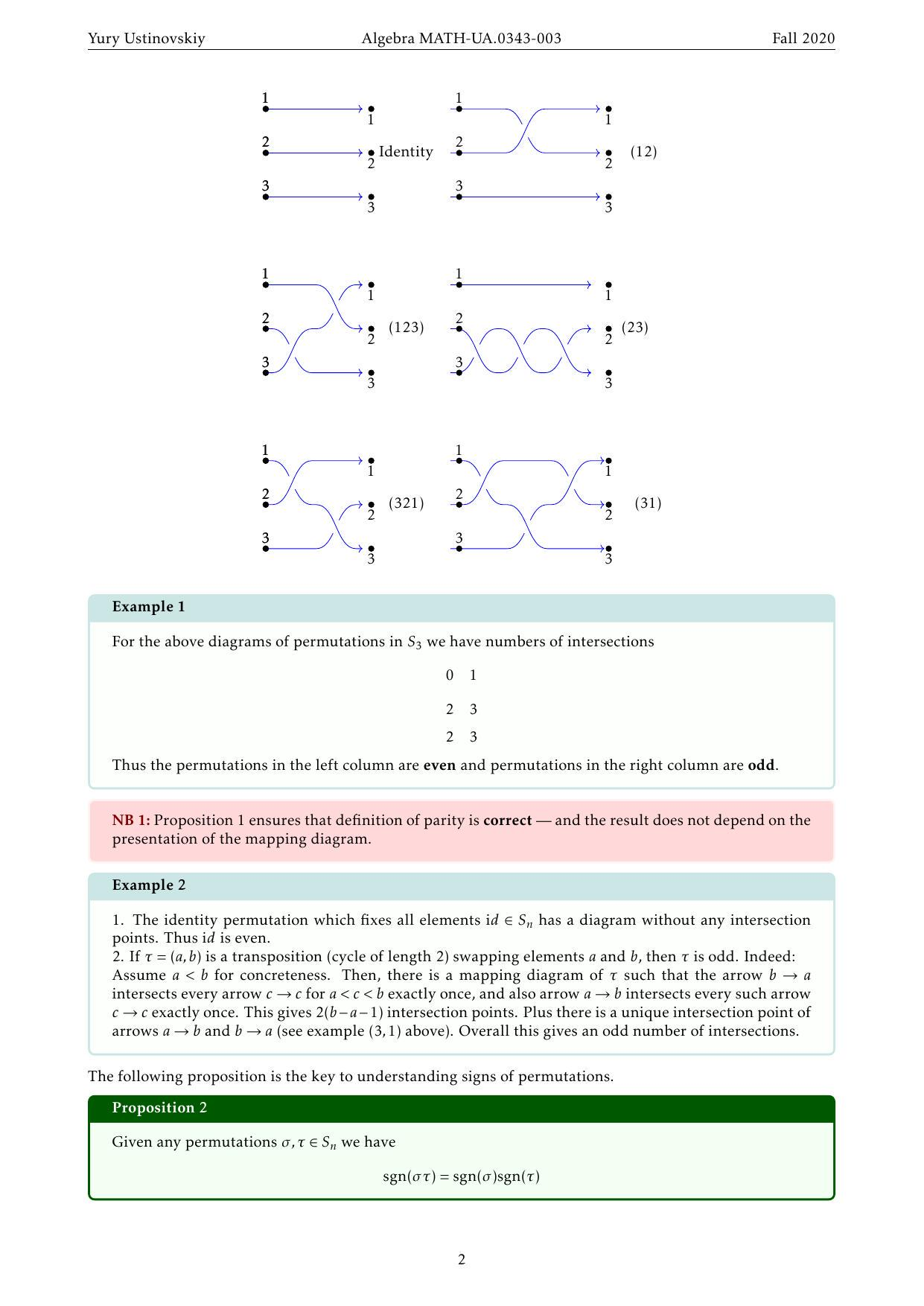# 抽象代数代写| Parity of permutations

1. Permutation group

2. Parity of permutation

On of the key characteristics of a permutation $\sigma \in S_{n}$ is its parity – it turns out that we can split all permutation into odd and even, and this splitting satisfies very nice properties.

Let $\sigma \in S_{n}$ be any permutation. Draw its mapping diagram. We allow arrows to move right/left as long as they always point into the bottom half-plane (below you can see 6 diagrams for $S_{3}$ going from top to bottom).

3. Proposition 1: Parity of intersection count

Given any $\sigma \in S_{n}$ consider its mapping diagram. Let $I$ be the number of intersection points of arrows ${ }^{a}$. Then for any other drawing of the mapping diagram with $J$ intersection points, we will have

$$I=J \bmod 2 .$$

i.e. $I$ and $J$ have the same parity

${ }^{a}$ To be pedantic: we assume that arrows are smoth curves, and they are not tangent at the intersection points.

Proof. The reason why parity of $I$ does not change, when we move arrow around is best explained in the following picture

This picture shows that intersection points appear and disappear in pairs, thus the parity of the number of the intersection points does not change.

4. Definition 1: Parity \& sign of permutation

Consider any mapping diagram of a permutation $\sigma \in S_{n} .$ Let $I$ be the number of intersection points of the arrows. The parity of permutation $\sigma$ is the parity of $I$.

Equivalently, we can express the parity of permutation in terms of its sign $\operatorname{sgn}(\sigma) \in{-1,+1} .$ Namely, if $\sigma$ is even, we will say that $\operatorname{sgn}(\sigma)=1$, and if $\sigma$ is odd, we will say $\operatorname{sgn}(\sigma)=-1 .$5. Example 1

For the above diagrams of permutations in $S_{3}$ we have numbers of intersections

$$01$$

\begin{aligned} & 23 \ & 23 \end{aligned}

Thus the permutations in the left column are even and permutations in the right column are odd.

NB 1: Proposition 1 ensures that definition of parity is correct – and the result does not depend on the presentation of the mapping diagram.

6. Example 2

1. The identity permutation which fixes all elements id $\in S_{n}$ has a diagram without any intersection points. Thus id is even.
2. If $\tau=(a, b)$ is a transposition (cycle of length 2) swapping elements $a$ and $b$, then $\tau$ is odd. Indeed: Assume $a<b$ for concreteness. Then, there is a mapping diagram of $\tau$ such that the arrow $b \rightarrow a$ intersects every arrow $c \rightarrow c$ for $a<c<b$ exactly once, and also arrow $a \rightarrow b$ intersects every such arrow $c \rightarrow c$ exactly once. This gives $2(b-a-1)$ intersection points. Plus there is a unique intersection point of arrows $a \rightarrow b$ and $b \rightarrow a$ (see example $(3,1)$ above). Overall this gives an odd number of intersections.

The following proposition is the key to understanding signs of permutations.

7. Proposition 2

Given any permutations $\sigma, \tau \in S_{n}$ we have

$$\operatorname{sgn}(\sigma \tau)=\operatorname{sgn}(\sigma) \operatorname{sgn}(\tau)$$

Proof. If mapping diagram for $\tau$ has $I(\tau)$ intersection points, and mapping diagram for $\sigma$ has $I(\sigma)$ intersection points, then the mapping diagram for $\sigma \tau$ obtained from “stacking” diagrams for $\sigma$ and $\tau$ together, will have $I(\tau)+I(\sigma)$ intersection points. This implies the desired identity.

8. Remark 1

lternatively, you can think about the above claim as follows:

$$\text { product of even permutations is even }$$

\begin{aligned} & \text { product of odd permutations is even } \end{aligned}

product of an odd and an even permutations (in any order) is odd

9. Example 3

ycle $\mu=\left(a_{1}, \ldots, a_{k}\right)$ of length $k$ can be factored into a product of $(k-1)$ transpositions (Problem #2 from Homework #7). Let us call these transpositions $\tau_{i}$. Therefore

$$\operatorname{sgn}(\mu)=\operatorname{sgn}\left(\tau_{1}\right) \ldots \operatorname{sgn}\left(\tau_{k-1}\right)=(-1)^{k-1}$$

Thus $\mu$ is even if $k$ is odd, and vice versa $\mu$ is odd if $k$ is even.

Problem 1: Let us think of $S_{4}$ as the group of symmetries of a tetrahedron. Then each rigid motion of a tetrahedron can be classified as odd or even, according to the parity of the corresponding permutation. Give a geometric characterization distinguishing the odd rigid motions from the even.

10. Example 4

Consider permutation

$$\sigma=\left(\begin{array}{llllll} 1 & 2 & 3 & 4 & 5 & 6 \ 3 & 4 & 1 & 6 & 2 & 5 \end{array}\right)$$

Of course we could draw its diagram and try to find its parity by intersection points count. But a much more efficient approach relies on Proposition 2 and the factorization of $\sigma$ into independent cycles:

First we factorize $\sigma$ into cycles

$$\sigma=(1,3)(2,4,6,5)$$

Both cycles $(1,3)$ and $(2,4,6,5)$ are of even length, thus they have odd parity by the previous example. Therefore $\sigma$ as a product of two odd permutations is even itself.

Problem 2: Let $A_{n} \subset S_{n}$ be the subset consisting of all even permutations. Prove that $A_{n}$ is a subgroup.